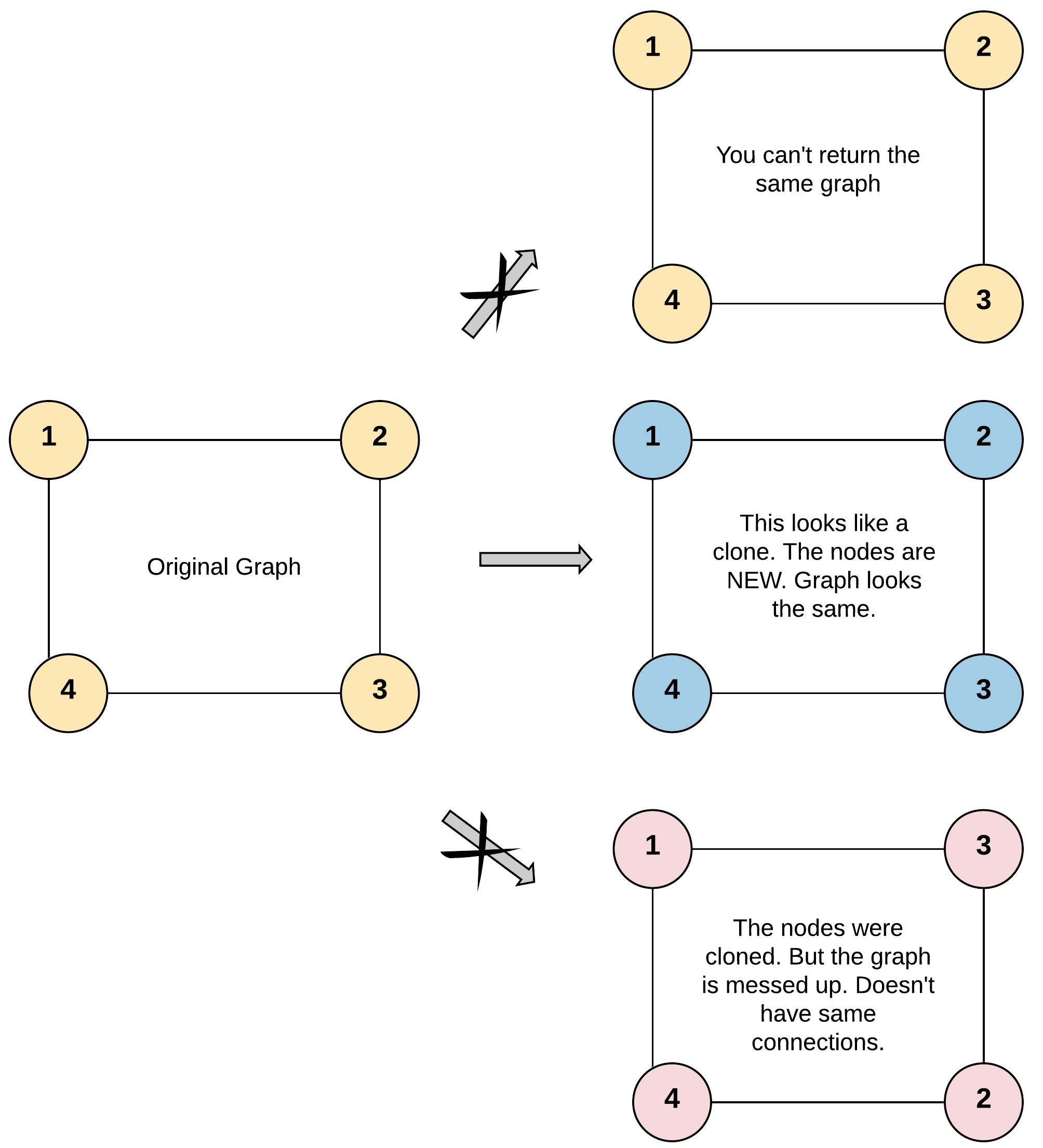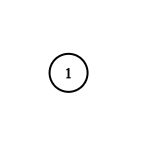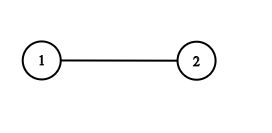# GeetCode Hub

Given a reference of a node in a connected undirected graph.

Return a deep copy (clone) of the graph.

Each node in the graph contains a value (`int`) and a list (`List[Node]`) of its neighbors.

```class Node {
public int val;
public List<Node> neighbors;
}
```

Test case format:

For simplicity, each node's value is the same as the node's index (1-indexed). For example, the first node with `val == 1`, the second node with `val == 2`, and so on. The graph is represented in the test case using an adjacency list.

An adjacency list is a collection of unordered lists used to represent a finite graph. Each list describes the set of neighbors of a node in the graph.

The given node will always be the first node with `val = 1`. You must return the copy of the given node as a reference to the cloned graph.

Example 1:```Input: adjList = [[2,4],[1,3],[2,4],[1,3]]
Output: [[2,4],[1,3],[2,4],[1,3]]
Explanation: There are 4 nodes in the graph.
1st node (val = 1)'s neighbors are 2nd node (val = 2) and 4th node (val = 4).
2nd node (val = 2)'s neighbors are 1st node (val = 1) and 3rd node (val = 3).
3rd node (val = 3)'s neighbors are 2nd node (val = 2) and 4th node (val = 4).
4th node (val = 4)'s neighbors are 1st node (val = 1) and 3rd node (val = 3).
```

Example 2:```Input: adjList = [[]]
Output: [[]]
Explanation: Note that the input contains one empty list. The graph consists of only one node with val = 1 and it does not have any neighbors.
```

Example 3:

```Input: adjList = []
Output: []
Explanation: This an empty graph, it does not have any nodes.
```

Example 4:```Input: adjList = [,]
Output: [,]
```

Constraints:

• The number of nodes in the graph is in the range `[0, 100]`.
• `1 <= Node.val <= 100`
• `Node.val` is unique for each node.
• There are no repeated edges and no self-loops in the graph.
• The Graph is connected and all nodes can be visited starting from the given node.

/* // Definition for a Node. class Node { public int val; public List<Node> neighbors; public Node() { val = 0; neighbors = new ArrayList<Node>(); } public Node(int _val) { val = _val; neighbors = new ArrayList<Node>(); } public Node(int _val, ArrayList<Node> _neighbors) { val = _val; neighbors = _neighbors; } } */ class Solution { public Node cloneGraph(Node node) { } }